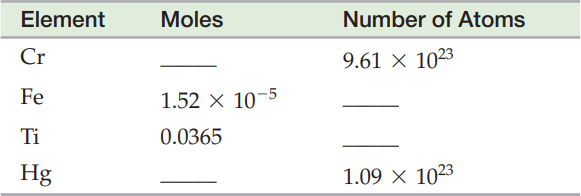×
Get Full Access to Introductory Chemistry - 5 Edition - Chapter 6 - Problem 22p
Get Full Access to Introductory Chemistry - 5 Edition - Chapter 6 - Problem 22p

×

# Complete the table.27. Element28. Moles29. Number of Atoms30. Cr31.32. 9.61 × 102333ISBN: 9780321910295 34

## Solution for problem 22P Chapter 6

Introductory Chemistry | 5th Edition

• Textbook Solutions
• 2901 Step-by-step solutions solved by professors and subject experts
• Get 24/7 help from StudySoup virtual teaching assistantsIntroductory Chemistry | 5th Edition

4 5 1 385 Reviews
19
1
Problem 22P

Problem 22P

Complete the table.

 27. Element 28. Moles 29. Number of Atoms 30. Cr 31. 32. 9.61 × 1023 33. Fe 34. 1.52 × KT5 35. 36. Ti 37. 0.0365 38. 39. Hg 40. 41. 1.09 × 1023

Step-by-Step Solution:

Problem 22P

Complete the table.Step by Step Solution

Step 1 of 6

The mole of a particular element constitutes theatoms and similar entities. This quantity reflects on the mass or the quantity of the compound.

The Avogadro’s number is employed to connect the number of atoms and the quantity of the compound created from those atoms.

Step 2 of 6

Step 3 of 6

##### ISBN: 9780321910295

Unlock Textbook Solution